# Suspension

Jump to: navigation, search
This article describes a construct that involves some variant of taking a product of a topological space with the unit interval and then making some identifications, typically at the endpoints, based on some specific maps.
View more such constructs

## Definition

### Long definition

Given a topological space$X$, the suspension of$X$, denoted$SX$, is defined as the quotient of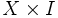$X \times I$ (where$I$ is the closed unit interval and we use the product topology) by the following two equivalence relations: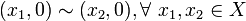$\! (x_1,0) \sim (x_2,0), \forall \ x_1,x_2 \in X$

and$\! (x_1,1) \sim (x_2,1) \ \forall \ x_1,x_2 \in X$

### Short definition

The suspension$SX$ of a topological space$X$ can be described in the following succinct ways as a quotient space$SX = (X \times [0,1]/(X \times \{ 0 \}))/(X \times \{ 1 \})$. In other words, we quotient out successively (or simultaneously) by the subspaces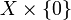$X \times \{ 0 \}$ and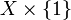$X \times \{ 1 \}$.

## In terms of other constructions

### Double mapping cylinder

The suspension can be viewed as a case of a double mapping cylinder where$Y$ and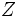$Z$ are both one-point spaces and both the maps involved send$X$ to the one point.

### Join

The suspension can also be viewed as the join of$X$ with the 0-sphere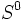$S^0$.

## Relation between a space and its suspension

### Homology for suspension

Further information: homology for suspension

Taking the suspension shifts the homology groups. Specifically, for any topological space$X$:$H_{k + 1}(SX) \cong H_k(X), \qquad k \ge 1$

The result extends to the zeroth homology if we use reduced homology instead of homology. (Without reduced homology, the formulation becomes more clumsy):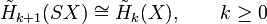$\tilde{H}_{k+1}(SX) \cong \tilde{H}_k(X), \qquad k \ge 0$

This result is an easy application of the Mayer-Vietoris homology sequence, and is similar to the application of the Seifert-van Kampen theorem to show that suspension of path-connected space is simply connected.